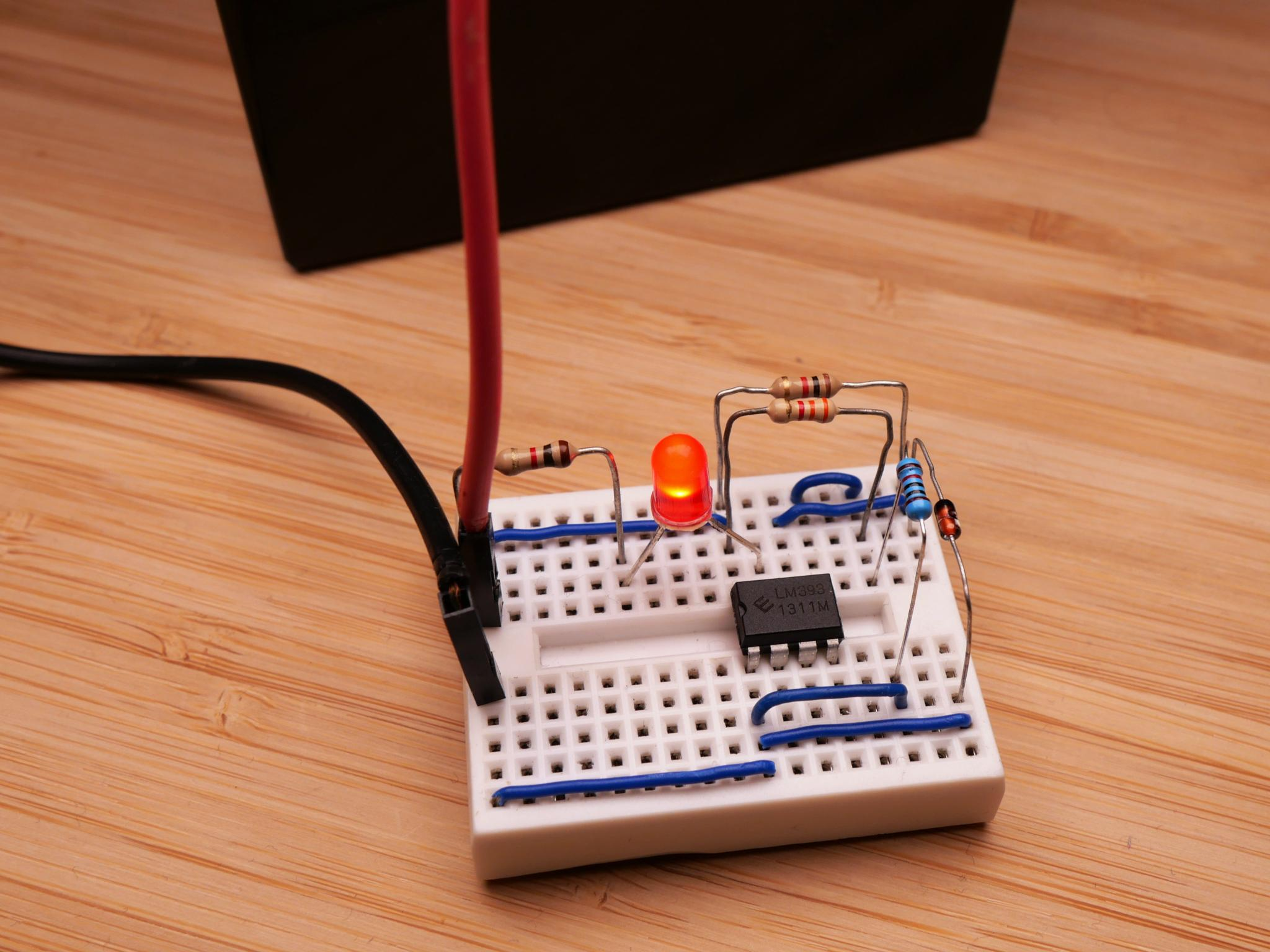# Zener Diode Low Voltage Alarm

Today, we are going to build a low voltage alarm using a zener diode and an LM393 comparator.

## Low Voltage Alarm with a Zener Diode

Last time, we looked at how a zener diode can be used as a voltage reference. This time, we are going to look at a more practical example. We are going to build a low voltage alarm for a 12 V lead acid battery using a zener diode and a comparator.

Let's have a look at what we are trying to achieve: we want an LED or a buzzer to turn on as soon as the battery voltage drops below a certain level. For that we have to define a suitable threshold value.

For a 12 V AGM lead acid battery, 12 V is a good value. That might seem surprising at first, but a modern 12 V AGM lead acid battery gets charged with 14.7 V and should have a voltage of roughly 13 V when fully charged. Additionally, it should not be discharged below 50 % of its capacity, if you want it to last for a long time. These 50 % happen to be reached at 12 V.

Enough theory. Let's build the circuit.

## The Circuit

For the circuit we again use the LM393 comparator. A comparator compares the voltages at its two inputs. In case the voltage at its non-inverting input (+) is lower than the one at the inverting input (-), its output becomes low and the LED turns on.

In the circuit I used a 9 V zener diode as a voltage reference and connected it to the LM393's inverting input.

How does that match our goal to turn the LED on at a battery voltage of 12 V?
For measuring the battery voltage, we are going to use a voltage divider. We need to choose the resistor values so that at 12 V the voltage divider outputs 9 V. This can be achieved by using a 3.3 kΩ and a 10 kΩ resistor. If you want to know more on how to calculate these values, I recommend you to have a look at my article about voltage dividers.

This is how the finished circuit looks like:## The Result

To use the circuit, connect it to the battery in parallel to the load. As soon as the battery voltage drops below 12 V, the LED will turn on.